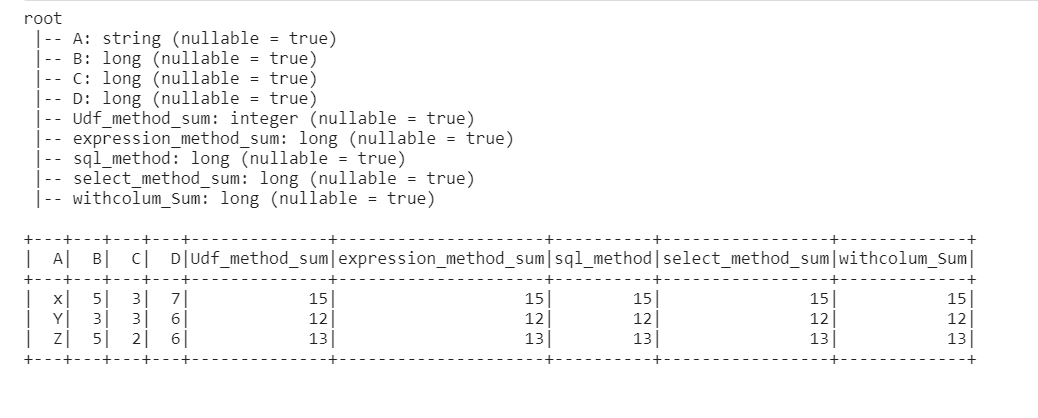Open In App

# How to add column sum as new column in PySpark dataframe ?

In this article, we are going to see how to perform the addition of New columns in Pyspark dataframe by various methods. It means that we want to create a new column that will contain the sum of all values present in the given row. Now let’s discuss the various methods how we add sum as new columns

But first, let’s create Dataframe for Demonstration

## Python3

 `# import SparkSession from the pyspark``from` `pyspark.sql ``import` `SparkSession` `# build and create  the SparkSession``# with name "sum as new_col"``spark ``=` `SparkSession.builder.appName(``"sum as new_col"``).getOrCreate()` `# Creating the Spark DataFrame``data ``=` `spark.createDataFrame([(``'x'``, ``5``, ``3``, ``7``),``                              ``(``'Y'``, ``3``, ``3``, ``6``),``                              ``(``'Z'``, ``5``, ``2``, ``6``)],``                             ``[``'A'``, ``'B'``, ``'C'``, ``'D'``])` `# Print the schema of the DataFrame by``# printSchema()``data.printSchema()` `# Showing the DataFrame``data.show()`

Output: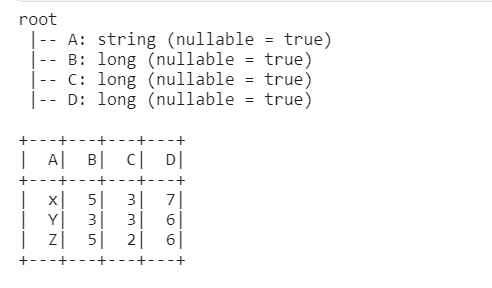Now we will see the different methods about how to add new columns in spark Dataframe .

### Method 1: Using UDF

In this method, we will define the function which will take the column name as arguments and return the total sum of rows. By using UDF(User-defined Functions) Method which is used to make reusable function in spark. This function allows us to create the new function as per our requirements that’s why this is also called a used defined function.

Now we define the datatype of the udf function and create the functions which will return the values which is the sum of all values in the row.

## Python3

 `# import the functions as F from pyspark.sql``import` `pyspark.sql.functions as F``from`  `pyspark.sql.types ``import` `IntegerType`` ` `# define the sum_col``def` `sum_col(b, c, d):``    ``col_sum ``=` `b``+``c``+``d``    ``return` `col_sum` `# integer datatype is defined``new_f ``=` `F.udf(sum_col, IntegerType())` `# calling and creating the new``# col as udf_method_sum``df_col1 ``=` `data.withColumn(``"Udf_method_sum"``,``                          ``new_f(``"B"``, ``"C"``, ``"D"``))` `# Showing and printing the schema of the Dataframe``df_col1.printSchema()``df_col1.show()`

Output: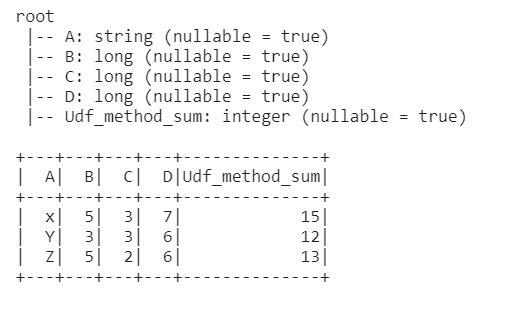### Method 2: Using expr() function.

By using expr(str) the function which will take expressions argument as a string. There is another function in pyspark that will take mathematical expression as an argument in the form of string. For example, if you want the sum of rows then pass the arguments as ‘n1+n2+n3+n4…….’ where n1,n2,n3… are the column names

## Python3

 `# import expr from the functions``from` `pyspark.sql.functions ``import` `expr` `# create the new column as by withcolumn``# by giving argument  as``# col_name ='expression_method_sum'``# and  expr() function which``# will take expressions  argument as string``df_col1 ``=` `df_col1.withColumn(``'expression_method_sum'``,``                             ``expr(``"B+C + D"``))` `# Showing and printing the schema of``# the Dataframe``df_col1.printSchema()``df_col1.show()`

Output: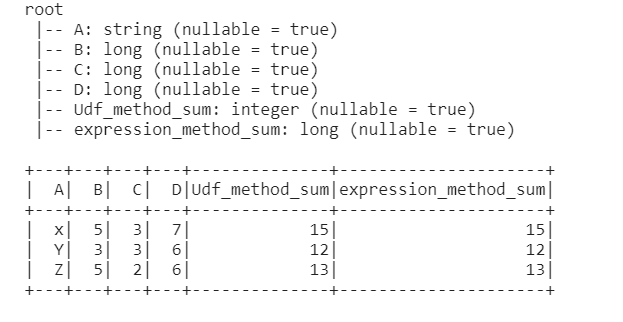### Method 3: Using SQL operation

In this method first, we have to create the temp view of the table with the help of createTempView we can create the temporary view. The life of this temp is upto the life of the sparkSession

Then after creating the table select the table by SQL clause which will take all the values as a string

## Python3

 `# Creating the temporary view``# of the DataFrame as temp.``df_col1 ``=` `df_col1.createTempView(``"temp"``)` `# By using sql clause creating``# new columns as sql_method``df_col1``=``spark.sql(``'select *, B+C+D as sql_method from temp'``)` `# Printing the schema of the dataFrame``# and showing the DataFrame``df_col1.printScheam()``df_col1.show()`

Output: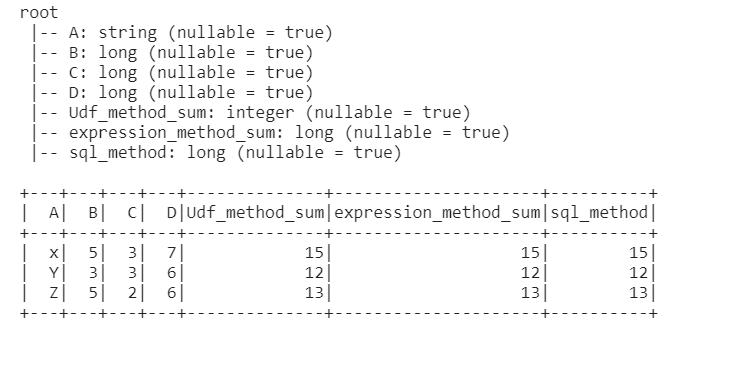### Method 4: Using select()

Select table by using select() method and pass the arguments first one is the column name , or “*” for selecting the whole table and the second argument pass the names of the columns for the addition, and alias() function is used to give the name of the newly created column.

## Python3

 `# select everything from table df_col1 and``# create new sum  column as " select_method_sum".``df_col1 ``=` `df_col1.select(``'*'``,``                         ``(df_col1[``"B"``]``+``df_col1[``"C"``]``+``df_col1[``'D'``]).``                         ``alias(``"select_method_sum"``))` `# Showing the schema and table``df_col1.printSchema()``df_col1.show()`

Output: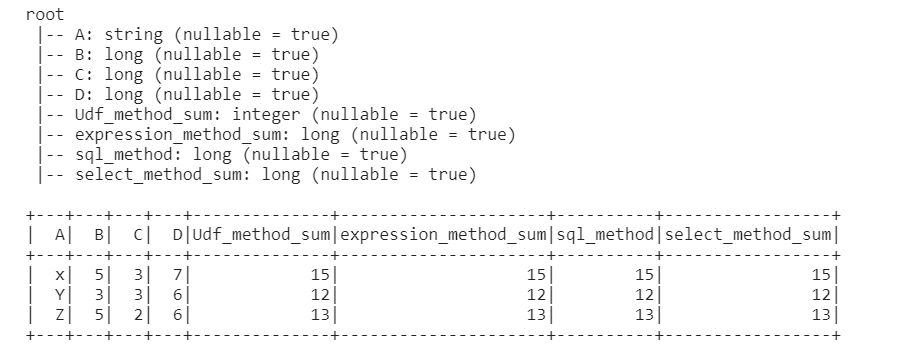### Method 5: Using withcolumn()

WithColumn() is a transformation function of the dataframe which is used for changing values, change datatypes, and creating new columns from existing ones.

This function will arguments as new column name and column name for the summation.

## Python3

 `# by using withcolumn function``df_col1 ``=` `df_col1.withColumn(``'withcolum_Sum'``,``                             ``data[``'B'``]``+``data[``'C'``]``+``data[``'D'``])` `# Showing and printing the schema``# of the Dataframe``df_col1.printSchema()``df_col1.show()`

Output: